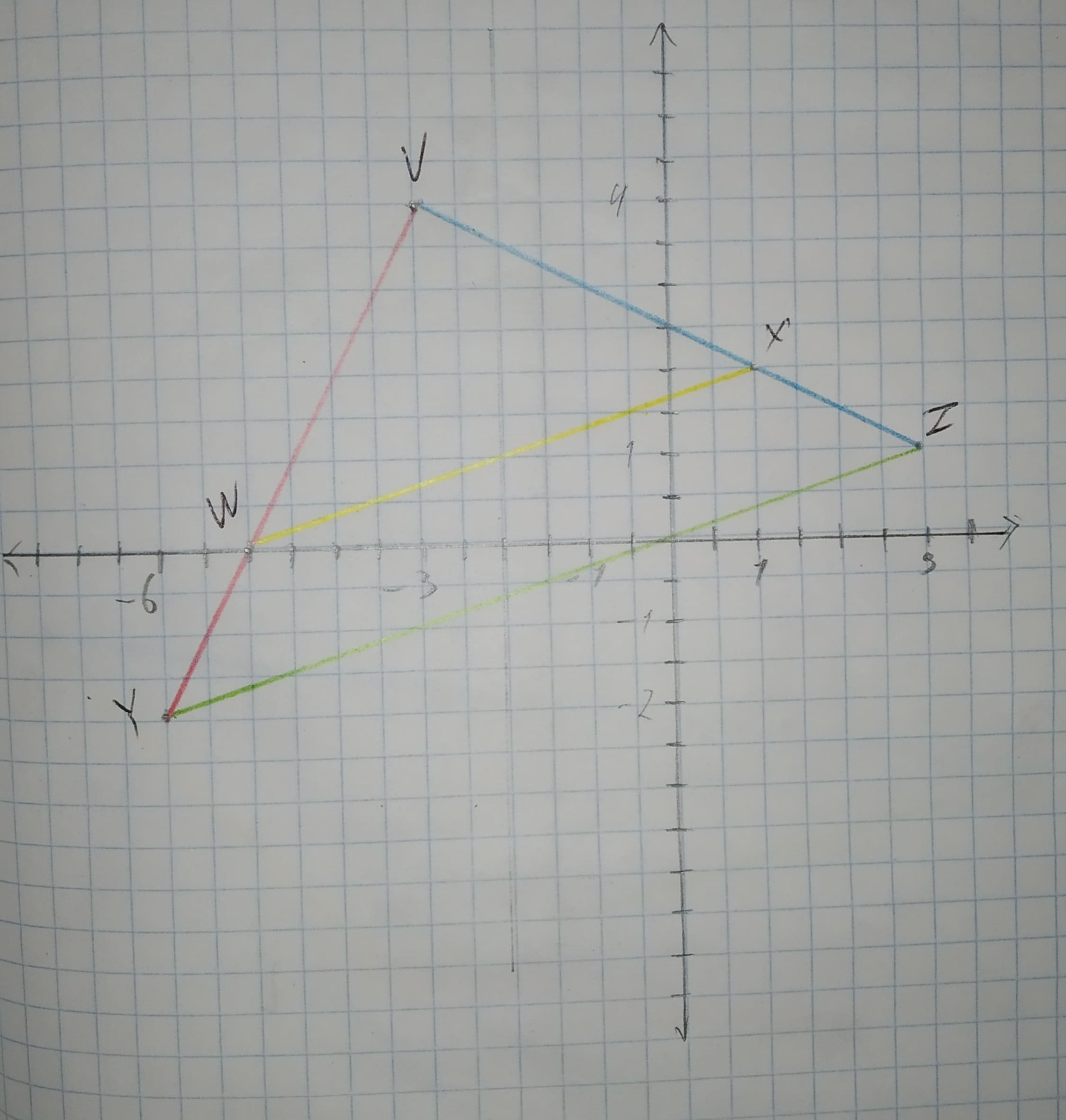# Draw a graph for the original figure and its dilated image. Check whether the dilation is a similarity transformation or not. Given: The given vertices are V(-3,4),W(-5,0),X(1,2),Y(-6,-2),Z(3,1)midtlinjeg 2020-11-20 Answered
Draw a graph for the original figure and its dilated image. Check whether the dilation is a similarity transformation or not.
Given:
The given vertices are
V(-3,4),W(-5,0),X(1,2),Y(-6,-2),Z(3,1)
You can still ask an expert for help

• Questions are typically answered in as fast as 30 minutes

Solve your problem for the price of one coffee

• Math expert for every subject
• Pay only if we can solve itfirmablogF

Calculation:
The graph for the given points V(-3,4),W(-5,0),X(1,2),Y(-6,-2),Z(3,1) is given below.Find the distance(using distance formula)of corresponding sides and find the ratio.
$VY=\sqrt{{\left[-3-\left(-6\right)\right]}^{2}+{\left[4-\left(-2\right)\right]}^{2}}=\sqrt{45}=3\sqrt{5}$
$VW=\sqrt{{\left[-3-\left(-5\right)\right]}^{2}+{\left(4-0\right)}^{2}}=\sqrt{20}=2\sqrt{5}$
$\frac{VW}{VY}=\frac{2\sqrt{5}}{3\sqrt{5}}=\frac{2}{3}$
$VZ=\sqrt{{\left(-3-3\right)}^{2}+{\left(4-1\right)}^{2}}=\sqrt{45}=3\sqrt{5}$
$VX=\sqrt{{\left(-3-1\right)}^{2}+{\left(4-2\right)}^{2}}=\sqrt{20}=2\sqrt{5}$
$\frac{VX}{VZ}=\frac{2\sqrt{5}}{3\sqrt{5}}=\frac{2}{3}$
$\mathrm{\angle }V\stackrel{\sim }{=}\mathrm{\angle }V$
From the above result, the lenght of the sides are proportional and the angles between them are congruent.
By the use of SAS similarity,
$\mathrm{△}ADE\sim \mathrm{△}ABC$.
Hence the dilation is a similarity transformation.